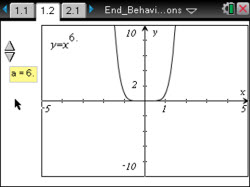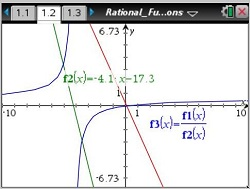### Crossing the Asymptote

This lesson involves determining when the graph of a rational function crosses its horizontal asymptote.
•TI-Nspire™ CX/CX II
•TI-Nspire™ CX CAS/CX II CAS
• TI-Nspire™ Navigator™
• TI-Nspire™
• TI-Nspire™ CAS### End Behavior of Polynomial Functions

Students will use a slider to scroll through the graphs of power functions with a coefficient of positive and negative 1 and determine similarities and differences among the functions. Students will generalize the end-behavior properties of various power functions.
•TI-Nspire™ CX/CX II
•TI-Nspire™ CX CAS/CX II CAS
• TI-Nspire™ Navigator™
• TI-Nspire™
• TI-Nspire™ CAS### Exploring Vertical Asymptotes

Students will be able to determine the domain of rational functions, use algebraic concepts to determine the vertical asymptotes of a rational function, determine the removable discontinuities of a rational function, and describe the graph of a rational function given the equation.
•TI-Nspire™ CX/CX II
•TI-Nspire™ CX CAS/CX II CAS
• TI-Nspire™ Navigator™### Multiplicity of Zeros of Functions

Students will utilize graphs and equations of five polynomial functions to determine the zeros of the functions and whether the functions cross the x-axis at these zeros or just touch the x-axis at the zeros. Then students will determine the degree of the polynomial functions and the effect the degree has upon the end behavior of the functions.
•TI-Nspire™ CX/CX II
•TI-Nspire™ CX CAS/CX II CAS
• TI-Nspire™ Navigator™
• TI-Nspire™
• TI-Nspire™ CAS### Polynomial Rollercoaster

This lesson involves finding a cubic regression equation to model a section of roller coaster track.
•TI-Nspire™ CX/CX II
•TI-Nspire™ CX CAS/CX II CAS
• TI-Nspire™ Navigator™
• TI-Nspire™
• TI-Nspire™ CAS### Products of Linear Functions

This lesson involves polynomial functions viewed as a product of linear functions.
•TI-Nspire™ CX/CX II
•TI-Nspire™ CX CAS/CX II CAS
• TI-Nspire™ Navigator™
• TI-Nspire™
• TI-Nspire™ CAS••